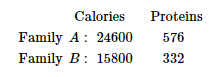# There are 2 families A and B. There are 4 men, 6 women and 2 children in family A, and 2 men, 2 women and 4 children in family B.

Question:

There are 2 families A and B. There are 4 men, 6 women and 2 children in family A, and 2 men, 2 women and 4 children in family B. The recommend daily amount of calories is 2400 for men, 1900 for women, 1800 for children and 45 grams of proteins for men, 55 grams for women and 33 grams for children. Represent the above information using matrix. Using matrix multiplication, calculate the total requirement of calories and proteins for each of the two families. What awareness can you create among people about the planned diet from this question?

Solution:

According to the question,

Let $X$ be the matrix showing number of family members in family $A$ and $B$.

$X=\left[\begin{array}{lll}4 & 6 & 2 \\ 2 & 2 & 4\end{array}\right]$ Family $B$

And, $Y$ be a matrix showing the recommend daily amount of calories.

$Y=\left[\begin{array}{l}2400 \\ 1900 \\ 1800\end{array}\right]$

And, $Z$ be a matrix showing the recommend daily amount of proteins.

$Z=\left[\begin{array}{l}45 \\ 55 \\ 33\end{array}\right]$

Now, the total requirement of calories of the two families will be shown by $X Y$.

$X Y=\left[\begin{array}{lll}4 & 6 & 2 \\ 2 & 2 & 4\end{array}\right]\left[\begin{array}{l}2400 \\ 1900 \\ 1800\end{array}\right]$

$=\left[\begin{array}{c}9600+11400+3600 \\ 4800+3800+7200\end{array}\right]$

$=\left[\begin{array}{c}24600 \\ 15800\end{array}\right]$

Also, the total requirement of proteins of the two families will be shown by $X Z$.

$X Z=\left[\begin{array}{lll}4 & 6 & 2 \\ 2 & 2 & 4\end{array}\right]\left[\begin{array}{l}45 \\ 55 \\ 33\end{array}\right]$

$=\left[\begin{array}{l}180+330+66 \\ 90+110+132\end{array}\right]$

$=\left[\begin{array}{l}576 \\ 332\end{array}\right]$

Hence, the total requirement of calories and proteins for each of the two families is shown as: